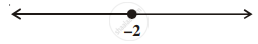# Solution of a linear inequality in variable x is represented on number line given below ______. - Mathematics

MCQ
Fill in the Blanks

Solution of a linear inequality in variable x is represented on number line given below ______.• x ∈ (–∞, –2)

• x ∈ (–∞, –2]

• x ∈ (–2, ∞]

• x ∈ [–2, ∞)

#### Solution

Solution of a linear inequality in variable x is represented on number line x ∈ (–∞, –2].
Explanation:

The given graph represents all real values of x less than and equal to –2.

So, x ∈ (–∞, –2].

Concept: Algebraic Solutions of Linear Inequalities in One Variable and Their Graphical Representation
Is there an error in this question or solution?
Chapter 6: Linear Inequalities - Exercise [Page 110]

#### APPEARS IN

NCERT Mathematics Exemplar Class 11
Chapter 6 Linear Inequalities
Exercise | Q 30 | Page 110
Share